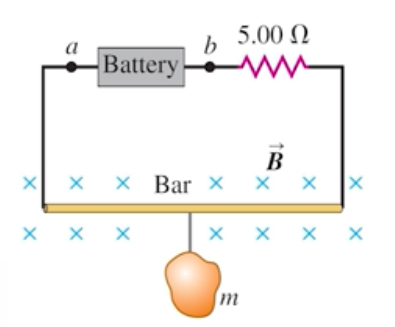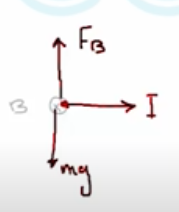Need Help?

Subscribe to Physics 2

###### \${selected_topic_name}
• Notes

$\begin{array}{l}{\text { An electromagnet produces a magnetic field of } 0.550 \mathrm{T}} \\ {\text { in a cylindrical region of radius } 2.50 \mathrm{cm} \text { between its poles. A }} \\ {\text { straight wire carrying a current of } 10.8 \mathrm{A} \text { passes through the center }} \\ {\text { of this region and is perpendicular to both the axis of the cylindri- - }} \\ {\text { cal region and the magnetic field. What magnitude of force is }} \\ {\text { exerted on the wire? }}\end{array}$

$F=(n A L)(q V_d B)=n q V_{2} A(L B)$

$= J A(L B)$

$F=IL B_{\perp}$

$=L I B \sin \phi=LIX B$

$F= IL B \sin \phi$

$L=0.05 \mathrm{m}$

$F=I L B=10.8 * 0.05 * 0.55=0.297 N$

$\begin{array}{l}{\text { The circuit shown in Fig. } \mathrm{E} 27.42 \text { is used to make a magnetic bal- }} \\ {\text { ance to weigh objects. The mass } m \text { to be measured is hung from }} \\ {\text { the center of the bar that is in a uniform magnetic field of } 1.50 \mathrm{T} \text { , }} \\ {\text { directed into the plane of the fig- ure. The battery voltage can be }} \\ {\text { adjusted to vary the current in the circuit. The horizontal bar is }} \\ {60.0 \mathrm{cm} \text { long and is made of extremely light-weight material. It is }} \\ {\text { connected to the battery by thin vertical wires that can support no }}\end{array}$

$\begin{array}{l}{\text { appreciable tension; all the weight of the suspended mass } m \text { is sup- }} \\ {\text { ported by the magnetic force on the bar. A resistor with } R=5.00 \Omega} \\ {\text { is in series with the bar; the resistance of the rest of the circuit is much }} \\ {\text { less than this. (a) Which point, } a \text { or } b \text { , should be the positive terminal }} \\ {\text { of the battery? ( b) If the maximum terminal voltage of the battery is }} \\ {175 \mathrm{V} \text { , what is the greatest mass } m \text { that this instrument can measure? }}\end{array}$$F_{B}=I L B \sin \phi$

$\rightarrow F_{B}=I L B$

$I=\frac{V}{R}$$F_{B}=m g \rightarrow I LB=m g$

$m=\frac{I L B}{g}=\frac{V L B}{R g}=\frac{175 * 0.6*1.5}{5 * 9.8}=3.21 \mathrm{kg}$